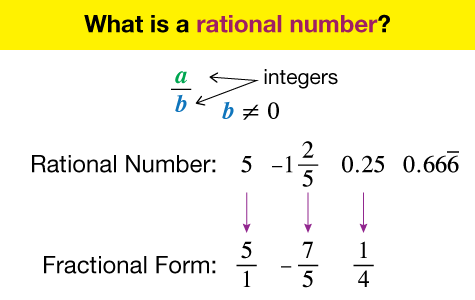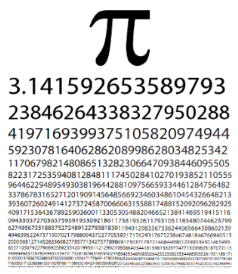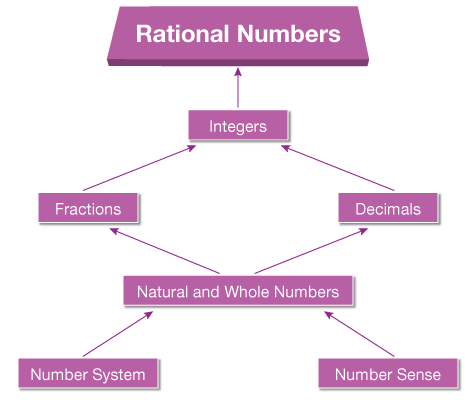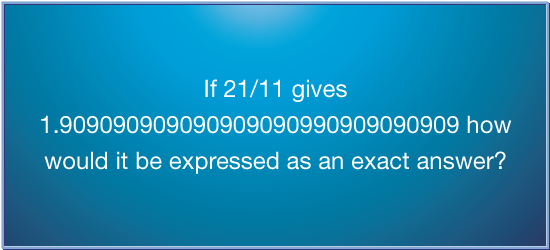# Rational Numbers

Go back to  'Maths'

## Introduction to Rational Numbers

So far you have studied about fractions, decimals, integers and even percentages. Well, now it’s time to expand your knowledge and understanding of the number system even further. Rational numbers have ended up being a challenge for the brightest of students because of the way they have been presented to them since the dawn of time. At Cuemath, we seek to change the dogmatic approach and utilize what has already been learnt to introduce new concepts.## The Big Idea: What are Rational Numbers?

Any number that can be represented in the form $$p \over q$$ where $$q$$ is not equal to zero, is called a Rational Number.

On reading this definition, one of the first questions that pop into our minds is, what are rational numbers? Well, think of all the numbers you have read about till today, in fact, let’s list them out shall we?

Integers, Fractions, Decimals, Whole numbers, Natural numbers, yes before we go any further, all these numbers are rational numbers.Why only a fraction? You may ask. We just listed a whole bunch of names of the numbers you have been introduced to, so then why limit ourselves to just fractions. Well, think about it;

Can $$5$$ be written as a fraction? Of course it can! It’s $${5 \over 1}$$ where the denominator is $$1$$ (non-zero). However, we deliberately didn’t use decimals in our description, it’s because while all fractions can be represented as decimals, not all decimals have a fractional representation. The square root of two and Pi are just two such examples.Rational numbers, like integers, fractions and decimals before it, also incorporates the four basic operations. The way these operations work is identical to what were followed for fractions. Click on the following link to get a more in-depth look at the operations using rational numbers:

## How do I understand?

### The Foundational Nature of Rational Numbers

Essentially, rational numbers represent the supergroup of all the different kinds of numbers that children have been exposed to thus far. It is very important that students develop a keen understanding of not only this topic, but also how this topic evolves and emerges from what has already been learnt.### How Do You Visualise Rational Numbers?

Remember the number line and how crucial a role it played in bridging the gap between numbers and their associated values and visualizing the same? Well, guess what, the notorious number line is going to be your best friend once again! Let’s take a look at what rational numbers look like on the number line!## Sub Topics

Here is link that will take you through the journey that every Cuemath students undertakes in the pursuit of understanding Rational Numbers along with practice worksheets:

## How do you Teach Rational Numbers?

Chunking: The secret behind mastering rational numbers lies in mastering the topics that lead up to it. So, when your child is practicing the application of rational numbers, feel free to throw in concepts belonging to integers, fractions and decimals. This builds your child’s ability to quickly recall previous concepts and also, allows them to appreciate the interconnectedness of mathematics as a subject.

Flash Cards: Flash cards are excellent learning tools. They keep you on your toes and can be pulled out at a moment’s notice for a quick dose of revision. Repeated practice and use of such flash cards keep concepts fresh in the mind of your child and ensure long term retention.Learn from the best math teachers and top your exams

• Live one on one classroom and doubt clearing
• Practice worksheets in and after class for conceptual clarity
• Personalized curriculum to keep up with school